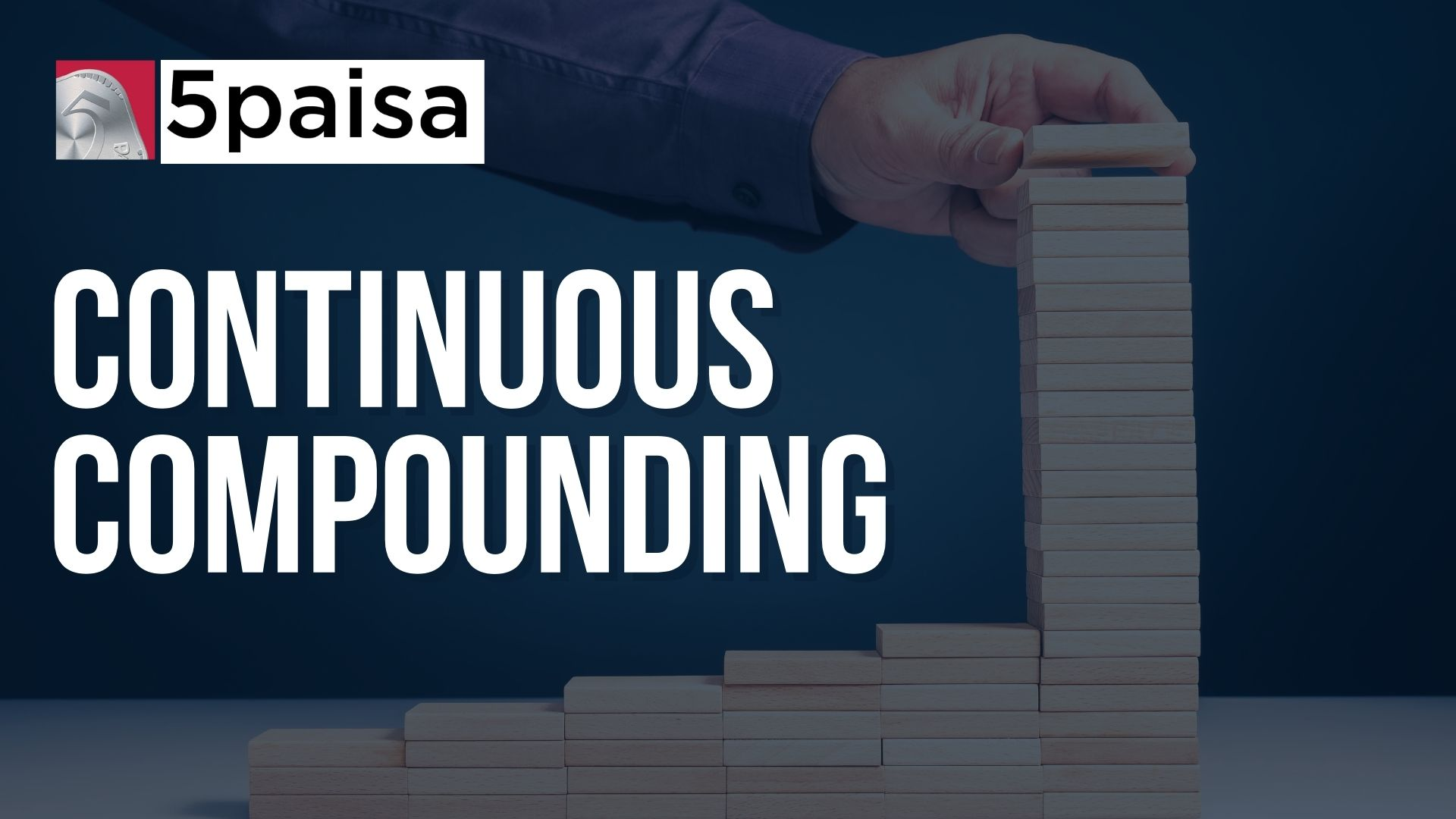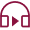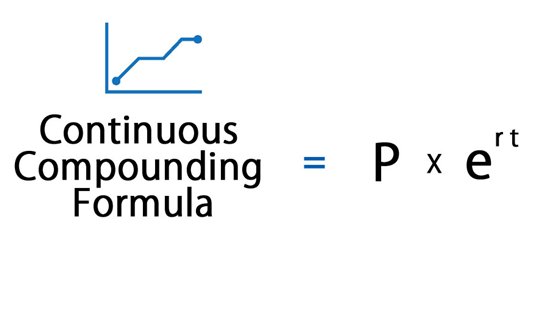# What is Continuous Compounding?What is Continuous Compounding?

Last Updated: May 09, 2023 - 05:27 pm 191 Views## Introduction

Continuous compounding is needed to calculate the rates of interest, which are crucial to running an economy. People look for the interest percentage before opening an FD account or investing in any portfolio, as the rate of interest is calculated using different means. One of the ways of calculating the interest is through continuous compounding. In this article, let’s understand what is continuous compounding and how you can calculate it.

## What is Continuous Compounding?

Continuous compounding is the limit of compound interest calculation where the interest is reinvested into an account’s balance an infinite number of times. It increases the interest component and the value of the portfolio of the entire investment. Although it may not be practical in the real world, it is essential in the financial world.

Additionally, it is a sporadic compounding case since most of the accrued interest is compounded monthly, quarterly, semi-annually, or annually. In simple words, continuous compounding assumes that interest is compounded and added back to the source account an infinite number of times. It means that an account is regularly earning interest, reinvesting the same interest back in the balance, and again earning interest on it.

## Importance of Continuous Compounding

Here are some of the reasons why continuous compounding is essential:

1.    It shows how much balance can be earned when the interest is accruing.
2.    It helps investors calculate how much they can expect from their investment, earning a continuously compounding interest.
3.    It further helps the investors to make a sound decision on where to reinvest this earned interest to make more profit.
4.    It also increases the amount faster than simple interest, as the latter is only calculated on the principal amount.
5.    Through compounding, money is multiplied at an increased rate. The greater the compounding periods, the more compound interest will be.
6.    It can also boost investment returns, specifically in the long run.

## Continuous Compounding Formula

Now that you have understood the continuous compounding meaning, it is time to look at its formula. Below is the compounded continuously formula:
A= Pert## Calculation of Compounding Formula

Here in this section, let’s understand the different elements of the continuous compound interest formula:
A= Pert

In the above,

A = The final amount
P = The initial amount
R = The interest rate
T = Time
E = the mathematical constant, where e = 2.7183

In this calculation, the time changes on how it is planned to be compounded. If it is quarterly, the time will be 1/4th.

If it is done bi-annually, the time will be 1/2th; if it is done annually, it would be 1/365.

The calculation can even happen on an hourly, minute, or daily basis as well. And in practical terms, that makes no value since the difference will only be in decimal points.

## How To Derive Continuous Compounding Formula?

The continuous compounding formula is derived from the compound interest formula. The compound interest formula is as below:
A = P (1 + r/n)nt

In the above,

A = the final amount or can also be called the future value
P = the initial amount
N = it is the number of times P or the initial amount is compounding
T = time
R = rate of interest

In the case of the continuous compound interest, n → ∞. So, the limit in the above formula is
A = limn→∞ P (1 + r/n)nt = Pert

Later on, in the final step, one of the limit formulas is used: limn→∞ (1 + r/n)n = er.

And from there, the continuous compounding formula is derived, which is

A= Pert

Examples Of How To Use Continuous Compounding
Let’s understand using the formula with the help of an example.

For example: The initial amount deposited in the bank is Rs. 2340 on a rate of interest: 3.1% on an annual basis. What will be the balance after three (3) years?

So, the formula says, A= Pert

From the above example, P = 2340,
r = 3.1, which will be 3.1/100 = 0.031
t = 3 (since we need to calculate for 3 years)
e = Napier's number, which is approximately 2.7183

Let’s calculate:
A = 2340 e0.031(3) ≈ 2568.06

So, after three years, the amount received will be Rs. 2568.05

Simple Interest v/s Compound Interest
There is a difference in how both these are calculated.

Simple interest is the interest received on the initial principal amount after a fixed term. In such cases, the interest is not added to the initial amount. The interest is paid on the initial amount at a specified time.

While on the other hand, in Compound interest, the initial principal amount changes to accommodate the earned interest. So every year, the amount you will receive will have the previous year's interest added to the initial principal amount.

## Conclusion

The formula checks the earned interest on the initial principal amount. In this case, the compounding time is often considered as infinity. Although continuous compounding shows the final value of the amount to be higher than the simple interest, it is challenging to apply in real-world cases. Therefore, it may not be too practical to use in real life as it holds significant importance in the financial world.

Instead, it holds value from the investor's end. Investors can check the amount they will receive on an ‘X’ investment and decide on future investment plans.

or

### Start Investing in 5 mins*

Rs. 20 Flat Per Order | 0% Brokerage

Tanushree is a seasoned professional with 6 years of experience in the Fintech and Edtech industry.

### Disclaimer

Investment/Trading in securities Market is subject to market risk, past performance is not a guarantee of future performance. The risk of loss in trading and investment in Securities markets including Equites and Derivatives can be substantial.

## Open Free Demat Account

Resend OTP
Account belongs to

By proceeding, you agree to the T&C.

Latest Blogs
Nifty Outlook for 9 June 2023

Our markets started the day on a positive and continued the positivity in the first hour of the trade. Nifty rallied towards 18800 but fell short to reach the level and it witnessed selling in the later part of the day. The index finally ended the day below 18650 with a loss of about half a percent.

• Jun 08, 2023
Nifty Outlook for 8 June 2023

Post consolidating in a range for the last few days, Nifty resumed its uptrend and rallied higher led by broader market participation. The index surpassed the 18700 mark and ended well above that with gains of around seven-tenths of a percent.

• Jun 07, 2023
Bearer Cheque

Introduction A bearer cheque is an efficient and adaptable financial tool. It is a method of payment where the cheque is made out to the owner or bearer of the document. Bearer cheques enable the holder to cash or deposit the cheque without any requirement for identification or endorsement, in contrast to other cheques payable to a specific person or business.

• Jun 06, 2023

### Start Investing Now!

Open Free Demat Account in 5 mins